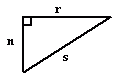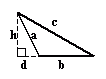Email us to get an instant 20% discount on highly effective K-12 Math & English kwizNET Programs!

#### Online Quiz (WorksheetABCD)

Questions Per Quiz = 2 4 6 8 10

### Grade 6 - Mathematics9.19 Classifying Triangles by Angles

#### Classification of triangles based on angles

1. Acute angled triangle:

• If all the angles of a triangle are acute angles, then it is called an acute angled triangle.

•2. Right angled triangle:
• A triangle in which one of the angles is a right angle is called a right angled triangle.

• In a right angled triangle, the side opposite to the right angle is called its hypotenuse.

•3. Obtuse angled triangle:
• A triangle containing an obtuse angle is called an obtuse angled triangle.

•Directions: Read the above properties of triangles and answer the following questions:
1. Illustrate each of the above properties by drawing a triangle.
2. Write in your own words, how to classify triangles according to their angles.

 Q 1: The angles 90 degrees, 30 degrees and 60 degrees represents an obtuse angled triangle.TrueFalse Q 2: In a triangle the angles are given by 55 degrees, 25 degrees and 100 degrees. The triangle is a (an) ________.Right angled scalene triangleAcute angled scalene triangleObtuse angled scalene triangle Q 3: The side opposite to the right angle in a right angled triangle is called the ________ of the triangle.Answer: Q 4: The angles 30 degrees, 60 degrees and 90 degrees represents a (an) ________ angled triangle.Answer: Question 5: This question is available to subscribers only! Question 6: This question is available to subscribers only!

#### Subscription to kwizNET Learning System offers the following benefits:

• Unrestricted access to grade appropriate lessons, quizzes, & printable worksheets
• Instant scoring of online quizzes
• Progress tracking and award certificates to keep your student motivated
• Unlimited practice with auto-generated 'WIZ MATH' quizzes